# 10. Canonical Transformations

### Point Transformations

It's clear that Lagrange's equations are correct for any reasonable choice of parameters labeling the system configuration. Let's call our first choice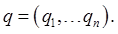Now transform to a new set, maybe even time dependent,The derivation of Lagrange's equations by minimizing the action still works, so Hamilton's equations must still also be OK too. This is called a point transformation: we've just moved to a different coordinate system, we're relabeling the points in configuration space (but possibly in a time-dependent way).

### General and Canonical Transformations

In the Hamiltonian approach, we're in phase space with a coordinate system having positions and momenta on an equal footing. It is therefore possible to think of more general transformations than the point transformation (which was restricted to the position coordinates).

We can have transformations that mix up position and momentum variables: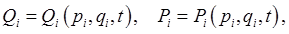where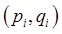means the whole set of the original variables.

In those original variables, the equations of motion had the nice canonical Hamilton form,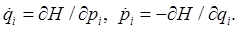Things won't usually be that simple in the new variables, but it does turn out that many of the "natural" transformations that arise in dynamics, such as that corresponding to going forward in time, do preserve the form of Hamilton's canonical equations, that is to sayA transformation that retains the canonical form of Hamilton's equations is said to be canonical.

(Jargon note: these transformations are occasionally referred to as contact transformations.)

### Generating Functions for Canonical Transformations

In this section, we go back to considering the full action (not the abbreviated--fixed energy--action used earlier).

Now, we've established that Hamilton's equations in the original parameterization follow from minimizing the action in the form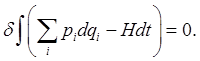For a canonical transformation, by definition the new variables must also satisfy Hamilton's equations, so, working backwards, action minimization must be expressible in the new variables exactly as in the old ones: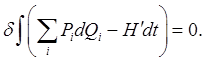Now, we've previously stated that two actions lead to the same equations of motion if the integrands differ by the total differential of some function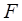of coordinates, momenta and time. (That's because in adding such a function to the integrand, the function's contribution to the integral is just the difference between its values at the two (fixed) ends, so in varying the path between the ends to minimize the total integral and so generate the equations of motion, this exact differential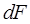makes no contribution.)

That is to say, the two action integrals will be minimized on the same path through phase space provided the integrands differ by an exact differential: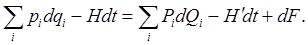is called the generating function of the transformation. Rearranging the equation above,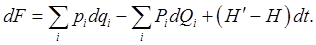Notice that the differentials here are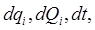so these are the natural variables for expressing the generating function.

We will therefore write it as, and from the expression forabove,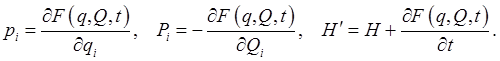Let's reemphasize here that a canonical transformation will in general mix up coordinates and momenta -- they are the same kind of variable, from this Hamiltonian perspective. They can even be exchanged: for a system with one degree of freedom, for example, the transformation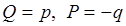is a perfectly good canonical transformation (check out Hamilton's equations in the new variables), even though it turns a position into a momentum and vice versa!

If this particular transformation is applied to a simple harmonic oscillator, the Hamiltonian remains the same (we're taking) so the differentialof the generating function (given above) has no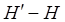term, it is just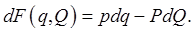The generating function for this transformation is easily found to be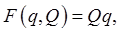from which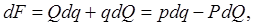as required.

Another canonical transformation for a simple harmonic oscillator is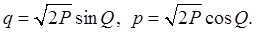You will investigate this in homework.

### Generating Functions in Different Variables

Thisis only one example of a generating function -- in discussing Liouville's theorem later, we'll find it convenient to have a generating function expressed in the's and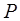's. We get that generating function, often labeledfromby a Legendre transformation:Then, for this new generating function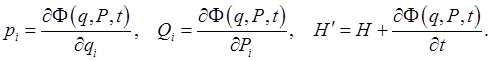.

Evidently, we can similarly use the Legendre transform to find generating functions depending on the other possible mixes of old and new variables: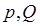, and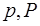.

### What's the Point of These Canonical Transformations?

It will become evident with a few examples: it is often possible to transform to a set of variables where the equations of motion are a lot simpler, and, for some variables, trivial. The canonical approach also gives a neat proof of Liouville's theorem, which we'll look at shortly.

### Poisson Brackets under Canonical Transformations

First, note that if Hamilton's equations have the standard canonical form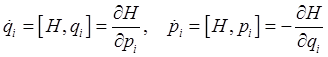with respect to a pair of variables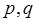then those variables are said to be canonically conjugate.

The Poisson bracket is invariant under a canonical transformation, meaning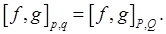Let's begin by establishing thatWe'll show the method by taking just one pair of variables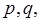and a generating function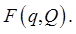Then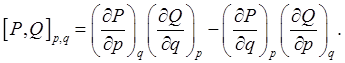With the generating functionwe have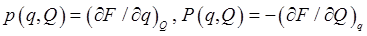, so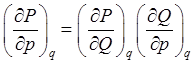and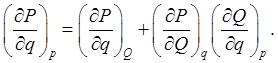Putting these results into the Poisson bracket,These basic results can then be used to prove the general Poisson bracket is independent of the parametrization of phase space, details in Goldstein and elsewhere.

Landau, on the other hand, offers a one-line proof of the invariance of the Poisson bracket of two dynamical functions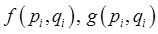under a canonical transformation: imagine a fictitious system having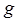as its Hamiltonian. Then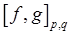is just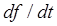, and cannot depend on the coordinate system used, so must equal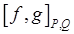.

### Time Development is a Canonical Transformation Generated by the Action

The transformation from the variables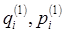at time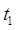toat a later timehas to be canonical, since the system obeys Hamilton's (canonical!) equations at all times.

In fact, the variation of the action along the true path from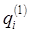at timetoatwith respect to final and initial coordinates and times was found earlier to be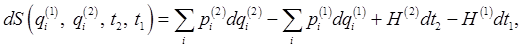and, comparing that expression with the differential form of a canonical transformation corresponding to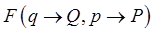in the discussion above, which waswe see that the action itself is the generating function for the canonical transformation from the variablesat timeto the set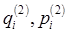at the later time, actuallygenerates the forward motion in time, the equivalent variables in the two equations above being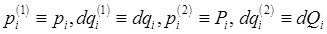.